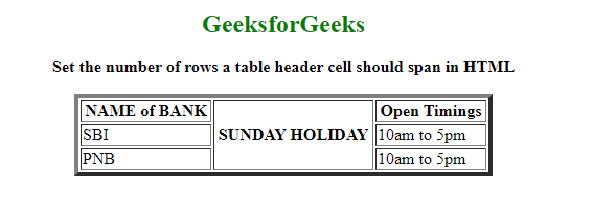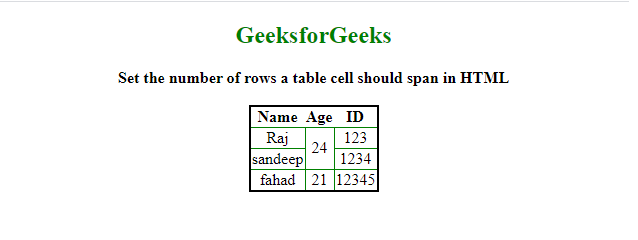# How to set the number of rows a table cell should span in HTML ?

• Last Updated : 26 May, 2021

In this article, we will show how multiple rows are spanned by a table header cell. The task can be done by using the rowspan attribute while using the <th> tag. This property is used to merge one or more cells as well as increase the height of the single header cell automatically.

Syntax:

`<th rowspan = "value">table content...</th>`

Example:

## HTML

 ` ` `<``html``> ` `  ``<``body``> ` `    ``<``center``> ` `      ``<``h2` `style``=``"color: green"``>GeeksforGeeks ` ` `  `      ``<``b``>  ` `       ``Set the number of rows a table header  ` `       ``cell should span in HTML  ` `      `` ` `      ``<``table` `border``=``"4"` `width``=``"400"``> ` `        ``<``tr``> ` `          ``<``th``>NAME of BANK ` `          ``<``th` `rowspan``=``"3"``>SUNDAY HOLIDAY ` `          ``<``th``>Open Timings ` `        `` ` ` `  `        ``<``tr``> ` `          ``<``td``>SBI ` `          ``<``td``>10am to 5pm ` `        `` ` ` `  `        ``<``tr``> ` `          ``<``td``>PNB ` `          ``<``td``>10am to 5pm ` `        `` ` `      `` ` `    `` ` `  `` ` ` `

Output:Example 2: In this example. we will learn, how rows can be made to span over multiple cells.

The task can be done by using the rowspan attribute when using the <td> tag within <tr> tag.  It allows the single table cell to span the height of more than one cell. It contains a numeric value that defines the number of rows that should be spanned.

Syntax:

```<tr>
<td rowspan = "value">table column content...</td>
</tr>```

## HTML

 ` ` `<``html``> ` `  ``<``head``> ` `    ``<``style``> ` `      ``table { ` `        ``border: 2px solid black; ` `        ``border-collapse: collapse; ` `        ``padding: 6px; ` `      ``} ` `      ``td { ` `        ``border: 1px solid green; ` `        ``text-align: center; ` `      ``} ` `    `` ` `  `` ` ` `  `  ``<``body` `style``=``"text-align: center"``> ` `    ``<``center``> ` `      ``<``h2` `style``=``"color: green"``>GeeksforGeeks ` `      ``<``b``>  ` `       ``Set the number of rows a table ` `       ``cell should span in HTML  ` `      `` ` ` `  `      ``<``br` `/> ` `      ``<``table``> ` `        ``<``tr``> ` `          ``<``th``>Name ` `          ``<``th``>Age ` `          ``<``th``>ID ` `        `` ` `        ``<``tr``> ` `          ``<``td``>Raj ` `          ``` `          ``<``td` `rowspan``=``"2"``>24 ` `          ``<``td``>123 ` `        `` ` `        ``<``tr``> ` `          ``<``td``>sandeep ` `          ``<``td``>1234 ` `        `` ` ` `  `        ``<``tr``> ` `          ``<``td``>fahad ` `          ``<``td``>21 ` `          ``<``td``>12345 ` `        `` ` `      `` ` `    `` ` `  `` ` ` ` `              `

Output:My Personal Notes arrow_drop_up
Recommended Articles
Page :